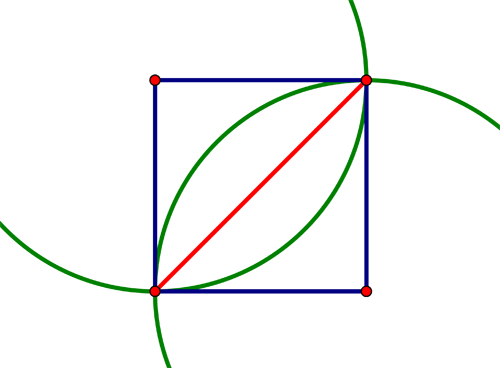SEARCH HOMEMath Central Quandaries & QueriesQuestion from Jean, a teacher: I have a square with side 4 cm. There are two overlapping arcs going from vertex to diagonal vertex. The other two vertices are the center of the arcs, which are shaded. How do I find the area of the shaded arcs? The overlapping arcs when shaded resemble a long thin football Thank you for your help.We have two responses for you

Hi Jean,In my diagram which is part of what you described, the red region is one-quarter of a circle of radius 4 cm. What is its area? What is the area of the green region?

Penny

Consider the figure below:One can calculate the two parts of the region each as the difference between a sector of the circle and the area of the triangle.

WalterMath Central is supported by the University of Regina and The Pacific Institute for the Mathematical Sciences.